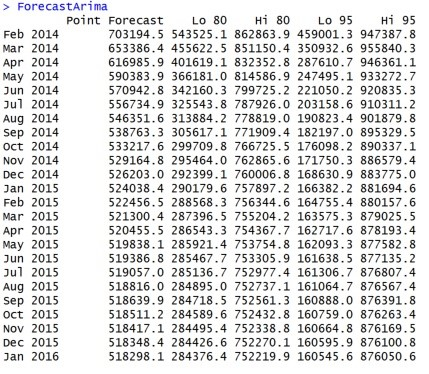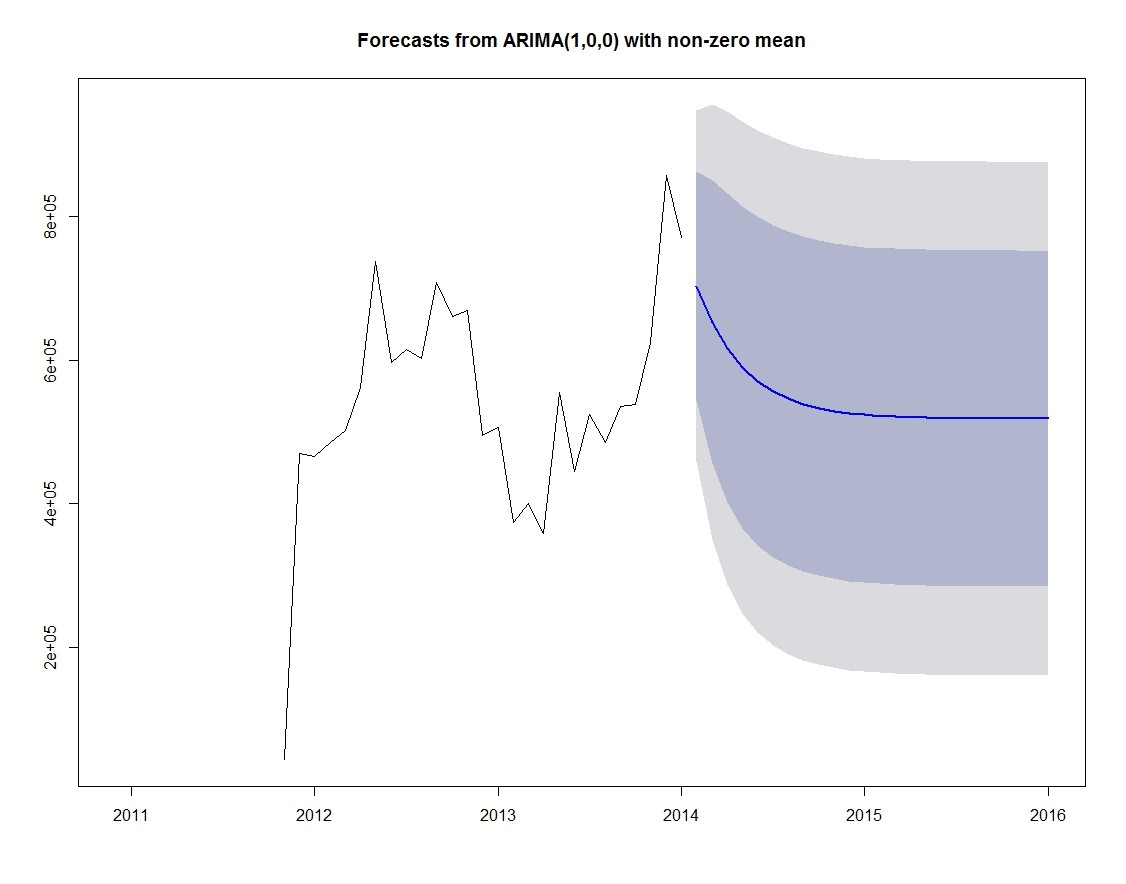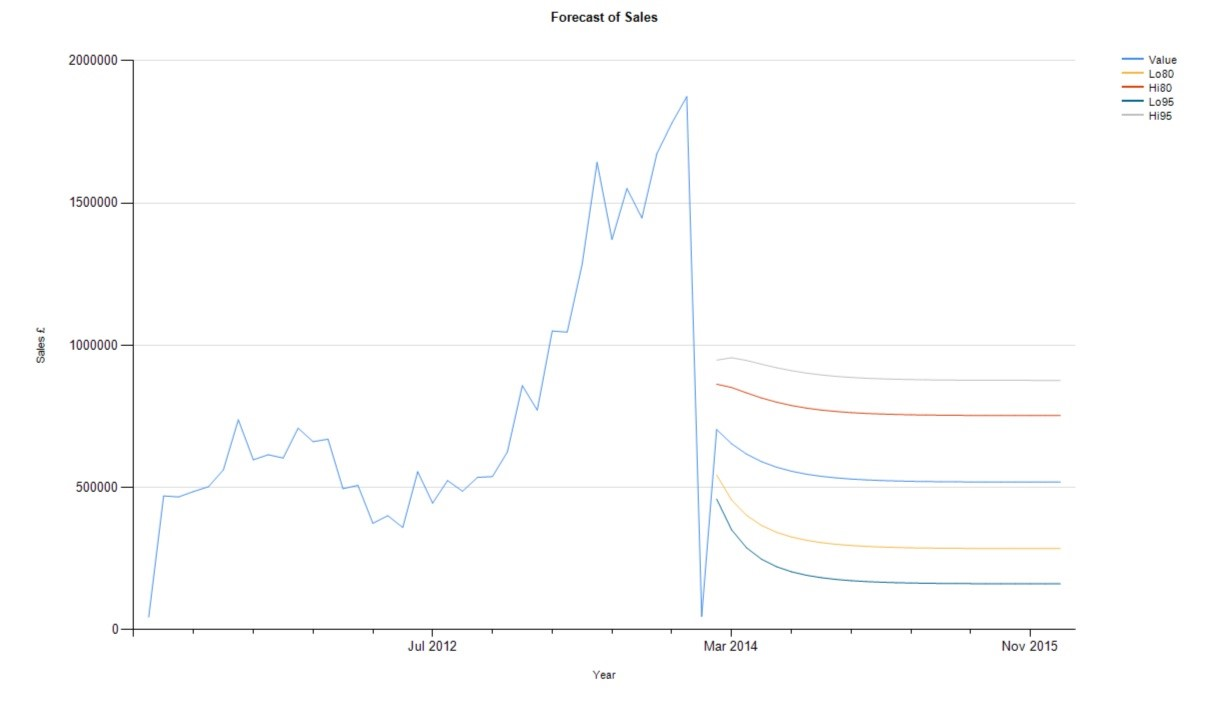# Forecasting: R and SQL Server 2016

Forecasting is pretty crucial to any business as nearly every function within a company needs an estimate of what the future will look like as a foundation to create and update plans. In a previous blog I addressed the principles and methods of forecasting and in this blog I will go through how you can forecast in R and SQL using the Autoregressive Integrated Moving Average (ARIMA) technique.

I will approach this in two parts. Part 1 will be demonstrating the forecast in an R platform; and in Part 2 I will be demonstrating the forecast using the R integration in SQL Server in conjunction with SSRS to visualise the results.

Part 1: The Pure R Approach

As mentioned, this will be creating a forecast using R and taking data from a SQL database. You will need to be able to connect SQL to R in order to do follow this section. You’ll also need to install the forecast CRAN package. As always we’ll be using AdventureWorks for the provision of data.

The R script is as follows:

library(RODBC)

library(forecast)

Data <- sqlQuery(SQLConnection, “WITH CTE AS(SELECT YearDate as[Year], , , , , , , , , , , ,   FROM (

SELECT * FROM (SELECT –DISTINCT

SUM(salesamount) as monthsales

,MONTH([OrderDate]) as monthdate

,YEAR(OrderDate) as  yeardate

group by MONTH(orderdate), year(orderdate)) t )

AS Table1

PIVOT(SUM(MonthSales) FOR Monthdate IN(,,,,,,,,,,,)) AS PVT

)

SELECT [Year], cast( as numeric(28,8)) as Jan, cast( as numeric(28,8)) as Feb, cast( as numeric(28,8)) as Mar, cast( as numeric(28,8)) as Apr, cast( as numeric(28,8)) as May,

cast( as numeric(28,8)) as Jun, cast( as numeric(28,8)) as Jul, cast( as numeric(28,8)) as Aug, cast( as numeric(28,8)) as Sep, cast( as numeric(28,8)) as Oct,

cast( as numeric(28,8)) as Nov, cast( as numeric(28,8)) as [Dec] FROM CTE

ORDER BY [Year] ASC“)

month <- c(1,2,3,4,5,6,7,8,9,10,11,12)

mn<- month.abb[month]

DataMonth <- Data[ ,mn]

DataTS<- ts(c(t(DataMonth)),start = c(2010,12), end = c(2014,1),frequency=12)

ForecastArima <- forecast(auto.arima(DataTS))

plot(ForecastArima)

ForecastArima

The numerical output will look like this:With the graphical output should look something like this:Part 2: Forecasting in SQL

Forecasting in SQL follows a pretty similar approach to the pure R approach in producing the numerical output. But that’s where the similarity ends. As we’ll want to visualise the output we’ll need to do some manipulation on the result from the R script we will have to wrap the initial query in a stored procedure which can be called by the stored procedure which will populate the graph in SSRS.

The first step is to write the initial stored procedure which will contain the R script

CREATE PROCEDURE dbo.spForecastSalesPreparation AS

BEGIN

EXECUTE sp_execute_external_script

@language = N’R’

,@script = N’library(forecast);

Data<- InputDataSet

month<- c(1,2,3,4,5,6,7,8,9,10,11,12)

mn<-month.abb[month]

DataMonth <- Data[ ,mn]

DataTS <- ts(c(t(DataMonth)), start = c(2010,12), end = c(2014,1), frequency =12)

ForecastArima <- forecast(auto.arima(DataTS))

,@input_data_1 = N’WITH CTE AS(SELECT YearDate as[Year],,,,,,,,,,,,  FROM (

SELECT * FROM (SELECT

SUM(salesamount) as monthsales

,MONTH([OrderDate]) as monthdate

,YEAR(OrderDate) as  yeardate

group by MONTH(orderdate), year(orderdate)) t )

AS Table1

PIVOT(SUM(MonthSales) FOR Monthdate IN(,,,,,,,,,,,)) AS PVT)

SELECT [Year],

cast( as numeric(28,8)) as Feb,

cast( as numeric(28,8)) as Mar,

cast( as numeric(28,8)) as Jan,

cast( as numeric(28,8)) as Apr,

cast( as numeric(28,8)) as May,

cast( as numeric(28,8)) as Jun,

cast( as numeric(28,8)) as Jul,

cast( as numeric(28,8)) as Aug,

cast( as numeric(28,8)) as Sep,

cast( as numeric(28,8)) as Oct,

cast( as numeric(28,8)) as Nov,

cast( as numeric(28,8)) as [Dec] FROM CTE

ORDER BY [Year] ASC’

WITH RESULT SETS ((“Point.Forecast” numeric(28,2) NOT NULL,

“Lo.80” NUMERIC(28,2) NOT NULL,

“Hi.80” NUMERIC(28,2) NOT NULL,

“Lo.95” NUMERIC(28,2) NOT NULL,

“Hi.95” NUMERIC(28,2) NOT NULL));

END;

The R script that you invoke is very similar to the one in the pure R approach, as is the SQL query. But the key element is the WITH RESULT SETS command, which returns the results of the query in a tabular format. Note, it is important to specify the data types for each of the columns you wish to return.

Because of the desire to visualise the results from R we’ll need to join the output from R with the input to R and keep it in a structure that makes sense, both as a table and as a graph. The stored procedure that I’ve created is as follows:

CREATE PROCEDURE dbo.spForecastSales AS

BEGIN

DECLARE @ForecastResults TABLE (Id INT IDENTITY(1,1),

Value NUMERIC(28,2),

Lo80 NUMERIC(28,2),

Hi80 NUMERIC(28,2),

Lo95 NUMERIC(28,2),

Hi95 NUMERIC(28,2))

DECLARE @FinalResults TABLE (Id INT IDENTITY(1,1),

ResultType VARCHAR(10),

YearDate INT,

MonthDate INT,

Value NUMERIC(28,2),

Lo80 NUMERIC(28,2),

Hi80 NUMERIC(28,2),

Lo95 NUMERIC(28,2),

Hi95 NUMERIC(28,2))

— insert the actual sales values

INSERT INTO @FinalResults (ResultType,

YearDate,

MonthDate,

Value)

SELECT       ‘Actual’

,YEAR(OrderDate)   as  YearDate

,MONTH(OrderDate)  as      MonthDate

,SUM(SalesAmount)  as    Value

FROM [dbo].[FactInternetSales]

GROUP BY MONTH(OrderDate), YEAR(OrderDate)

ORDER BY YearDate ASC, MonthDate ASC

— insert the forecast sales values

INSERT INTO @ForecastResults

EXECUTE dbo.spForecastSalesPreparation

DECLARE @MaxId INT,

@LastDate DATE;

SET @MaxId = (SELECT MAX(Id) FROM @FinalResults)

SELECT @LastDate = DATEFROMPARTS(YearDate, MonthDate, 1)

FROM @FinalResults

WHERE Id = @MaxId

INSERT INTO @FinalResults (ResultType,

YearDate,

MonthDate,

Value,

Lo80,

Hi80,

Lo95,

Hi95)

SELECT ‘Forecast’,

YearDate = DATEPART(year, DATEADD(month, Id, @LastDate)),

MonthDate = DATEPART(Month, DATEADD(month, Id, @LastDate)),

Value,

Lo80,

Hi80,

Lo95,

Hi95

FROM @ForecastResults

ORDER BY Id ASC

SELECT  Id,

ResultType,

DATEFROMPARTs(YearDate, MonthDate, 1) AS YearDate2,

Value,

Lo80,

Hi80,

Lo95,

Hi95

FROM @FinalResults

END;

Following the normal steps of creating a report in SSRS using a stored procedure as a dataset your graph might look something like this:So, we’ve shown two similar yet different approaches to forecasting using the ARIMA method and utilising R and SQL. Hopefully this demonstrates how quick and easy it is to establish a rudimentary forecasting practice wherever you are.